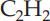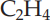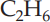×
Get Full Access to Introductory Chemistry - 5 Edition - Chapter 10 - Problem 72p
Get Full Access to Introductory Chemistry - 5 Edition - Chapter 10 - Problem 72p

×

# Determine the bond angles for each molecule in.(a) C2H2 (skeletal structure HCCH)(b)ISBN: 9780321910295 34

## Solution for problem 72P Chapter 10

Introductory Chemistry | 5th Edition

• Textbook Solutions
• 2901 Step-by-step solutions solved by professors and subject experts
• Get 24/7 help from StudySoup virtual teaching assistantsIntroductory Chemistry | 5th Edition

4 5 1 247 Reviews
15
3
Problem 72P

Problem 72P

Determine the bond angles for each molecule in.

(a) C2H2 (skeletal structure HCCH)

(b) C2H4 (skeletal structure H2CCH2)

(c)  C2H6 (skeletal structure H3CCH3)

Step-by-Step Solution:

Problem 72P

Determine the bond angles for each molecule in.

(a)(skeletal structure HCCH)

(b)(skeletal structure H2CCH2)

(c)(skeletal structure H3CCH3)

Step by Step Solution

Step 1 of 4

The hydrocarbons which contain single covalent bonds are named as alkanes. However, the unsaturated hydrocarbons which contain double or triple covalent bonds are regarded as an alkene and alkyne respectively.

Step 2 of 4

Step 3 of 4

## Discover and learn what students are asking

Calculus: Early Transcendental Functions : The Natural Logarithmic Function: Integration
?In Exercises 1-26, find the indefinite integral. $$\int \frac{1}{2 x+5} d x$$

Calculus: Early Transcendental Functions : Partial Derivatives
?In Exercises 7 - 38, find both first partial derivatives. $$z=y e^{y / x}$$

Unlock Textbook Solution

Enter your email below to unlock your verified solution to:

Determine the bond angles for each molecule in.(a) C2H2 (skeletal structure HCCH)(b)